## Annotation

When performing mathematical operations with infinite series, it is necessary to follow the rules of the positional number system.

## Number of terms

The number of terms of an infinite series, presented in the visible part of the series, must be considered as an analogue of writing a number using numbers in the positional number system. The number of terms in the visible part of the series is similar to the rounding of an ordinary decimal fraction with a certain accuracy, that is, to a certain number of decimal places.

When performing mathematical operations with infinite series, for each example it is necessary to use the same number of terms in the visible part of the series. If you do not follow this rule, it can lead to an erroneous result. In mathematics, it is not customary to use any number with different rounding accuracy in one example.

## Shifting series

Shifting an infinite series when performing mathematical operations automatically leads to an incorrect result. This is analogous to adding zeros to the decimal immediately after the decimal point.

Consider an example. If you subtract the same series without a shift from any series, the result will be zero. If the subtraction uses a shift and does not take into account the compensating group of terms, then the result will be different from zero. Suppose we have a series S. Let's write the series -S and add these two series. The result should be zero. By performing addition without shift, we get the correct result.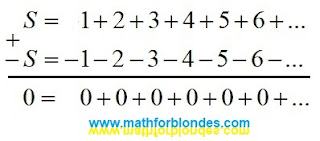Addition of infinite series without shift

Shift by one position leads to wrong result.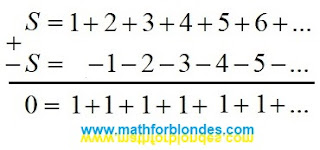Shift by one position

A two-position shift leads to another incorrect result.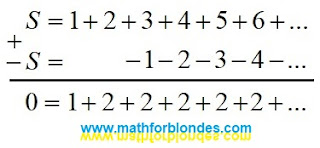A two-position shift

Shifts by an arbitrary number of positions allow you to get an infinite number of incorrect results.

Compliance with the rule about the same number of terms of an infinite series in one example and the use of the compensating group of terms (highlighted in curly brackets) avoids an error. But in the examples given, this method is more laborious than no shift.The use of a compensating group of terms

You can understand the reason for the occurrence of incorrect results if you consider the shift in arithmetic.

Let us analyze some of the most well-known examples of determining the sums of infinite divergent series.

## Sum of Grandi's series

In the given example, the sum of Grandi's series is equal to 1/2, which is not a correct result.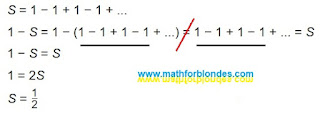Sum of Grandi's series

The second line contains an equals sign between two different sums: one sum consists of five terms that are not equal to zero, the second consists of four. In this example, the optical illusion of the equality of different sums is used, if one term is hidden behind the three-dots of infinity.

## The sum of the alternating series

Below is an alternating series and a solution for determining its sum.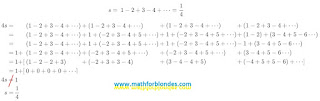The sum of the alternating series

In the proposed solution, the same series is represented by a different number of terms: from four to six. When performing mathematical operations and rearranging terms, the compensating group of terms is ignored. Two other methods for obtaining the sum 4s are not considered: addition of series without shift and multiplication of the original series s by 4. Both these methods give the same result, which indicates the mathematical accuracy of the calculations performed.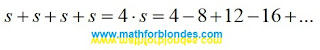The sum 4s

## Sum of natural numbers

Consider the sum of an infinite series of natural numbers. Intuitively, this is a divergent infinite series that cannot have a finite sum value. But, here is an example of calculating the sum of this infinite series.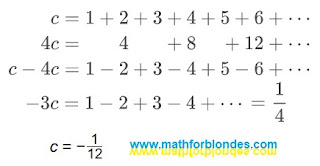Sum of natural numbers

Typical errors of these calculations are shown in the example above. Mathematicians did not check the solution: the original series c, multiplied by minus three, is equal to: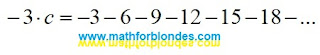Solution verification

## Conclusion

All the above ways of finding the sum of an infinite series are nothing more than fitting the solution to a given result. Nobody prevents mathematicians from establishing their own rules for virtual games in numbers. But the application of such results of "calculations" in the laws of physics leads to their misunderstanding. For example, a numerical coefficient due to some physical parameter or simply a correction factor is interpreted as the sum of some infinite series. An example of an erroneous interpretation of numerical coefficients would be the following statement: the area of a right triangle is equal to the sum of the Grandi series multiplied by the product of its legs.

## 8/12/2022

### Permutation of terms in infinite sums

From the school bench, everyone knows the rule that appeared in antiquity: the amount does not change from the rearrangement of the terms. By default, it is assumed that the number of terms and their value remain unchanged. If we change the number of terms or change the value of at least one of them, there can no longer be any talk of rearranging the terms. In this case, we have moved from one sum to another and these two sums are not connected in any way.

In the article “What is wrong with the rearrangement of terms?” (in Russian) an example of an infinite sum is given, allegedly proving that the final sum depends on the order of addition.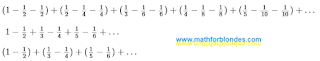Infinite sum

After opening the brackets and reducing the same terms, the result of the addition is different. That's what mathematicians say. Let's consider this example with more careful observance of the rules for writing mathematical expressions.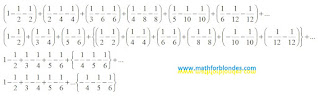Permutation of terms in infinite sums

The first line contains the original infinite sum, consisting of six brackets - three terms in each bracket. The total number of terms is 18, the total sum of the expression is zero. The entire mathematical expression can be divided into two groups: the visible part of the expression, represented by 18 terms, and the invisible part of the expression, consisting of an infinite number of brackets, three terms in each bracket. These two parts are separated by the three-dots of infinity. Both parts are zero.

The second line shows the same 18 terms after the permutation. The first three pairs of terms will be further presented in the visible part of the expression. Curly brackets highlight the compensating group of terms.

In the third line, the six terms of the visible part remain unchanged. The compensating group, after simplifying the expression, is represented by three terms. The final result after rearranging the terms has not changed and is still equal to zero.

The fourth line has nothing to do with mathematics. This is the usual trick of an illusionist who hid the compensating group of terms in the arm of infinity (the invisible part of the expression). The purpose of this trick is to convince gullible viewers of the “truthfulness” of the false statement about the change in the total amount after rearranging the terms. Yes, this is what card cheaters do - they hide the card in their sleeve or take it out of there. In the circus, such a trick is called an illusionist's trick. In legal terms, this is called fraud.

We can also give a cruder example of a permutation of the terms in this expression. If terms with only one sign are shown in the visible part of the expression, the audience will inevitably have a question where the terms with opposite signs have gone, and this will clearly make it difficult to demonstrate the trick.

The permutation of terms in infinite sums clearly demonstrates the principle of communicating vessels. The first vessel is the visible part of the expression. The second vessel is the invisible part of the expression, which includes the compensating group of terms. The three-dots of infinity is the connecting pipe between the vessels. The final sum of the expression is the total volume of liquid in the two vessels. Since in our mathematical example the total sum is equal to zero, then in relation to communicating vessels, we consider the initial total liquid level in the vessels as zero of the relative coordinate system. A change in the number or size of terms in the visible part of the expression will lead to a change in the number or size of terms in the compensating group.

If the last expression is considered without an invisible compensating group of terms, then it will not be the result of a rearrangement of the terms, but a completely different infinite sum, containing only a part of the terms from the original expression and not connected with it in any way. The proof of this fact is the different total sum of the two expressions.

## 8/11/2022

### Multiplication by zero in physics

To finish talking about multiplication by zero, consider an example from physics. There, all laws are written using multiplication. But, unlike mathematicians, physicists understand at least a little what they are talking about.

And so, for simplicity, consider Ohm's law. The simplest option: multiplying current by resistance gives voltage. I specifically use the language and style of mathematicians to describe a physical law. After all, we are trying to understand mathematics. Let's write this in physical notation.

I * R = U

Next, we write a mathematical example of multiplication.

a * b = c

Now find four differences. We have only the appearance of the three letters is different, everything else is the same. If one of the mathematicians begins to assert that the first expression has nothing to do with the second, then I have a question: why is such mathematics needed? Only for cramming? Multiplication perfectly describes physical laws, if by multiplication we mean interaction.

As a result of the interaction of current with resistance, a voltage is obtained. Electricity is given to us by a power plant. Resistance is provided by wires with a light bulb inside the room. The interaction of these physical quantities gives a voltage, which is expressed in the radiance of a light bulb. If there is current and wires, then there is voltage - the bulb is on.Multiplication by zero in physics

Consider the case where multiplication does not occur. The neighbor has electricity, we have wires, but we have no voltage. The light does not burn because the neighbor's current does not interact with our wires and the light bulb. This sad fact is similar to zero in formulas.

I and R = 0

a and b = 0

Now we will consider two cases of multiplication by zero. In the first case, everything is connected correctly, but the power plant does not work. As a result, the lamp does not light up. There is no interaction, no multiplication occurs.

0 * R = 0

0 * b = 0

In the second case, the power plant is working, but we do not have a light bulb. There is no interaction between resistance and current. Again, no multiplication occurs.

I * 0 = 0

a * 0 = 0

As an example from physics shows, multiplication by zero does not occur. The sacred definition of mathematicians "any number multiplied by zero equals zero" is no more than a shaman's lament while dancing with a tambourine.

### Multiplication by zero in geometry

Consider multiplication by zero in geometry. Multiplying the length by the width gives the area. Whatever mathematicians tell you, all the formulas for the areas of any geometric shapes come down to this simple formula. It’s just that for different figures there are different correction factors, but we’ll talk about this some other time.

We have a rectangle and a formula to calculate its area. Traditionally, it is portrayed as follows.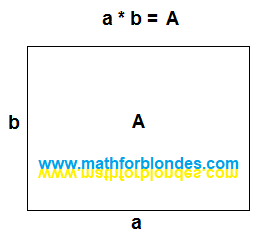Rectangle area

Everything seems to be correct. But... In algebra, we first write down the two factors, then we write the equal sign, and only after that we write down the result of the multiplication. This is a whole series, not just one picture. Let's represent geometrically everything that we write down algebraically. The sides of the rectangle a and b are line segments. The mathematical operation of multiplication has no geometric representation. I will replace the equal sign with a vertical line that divides the picture into two parts - before multiplication and the result of multiplication. The area of rectangle A is, in fact, the rectangle itself. Here's what happened.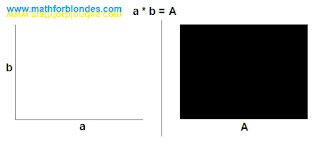Multiplication in geometry

Two perpendicular segments before multiplication turn into the area of a rectangle after multiplication. Now let's draw multiplication by zero.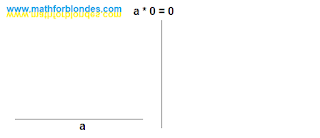Multiplication by zero

As a result of multiplication by zero, we do not get the area. We have the length, but the width is missing. Naturally, the area is simply nowhere to come from. We look at the result of multiplying zero.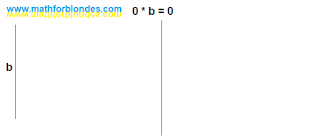Multiplying zero

Now we have a width but no length. Again, the area can not be obtained. Next, we depict the multiplication of zeros - zero multiplied by zero equals zero.Multiplication of zeros

No length, no width, no area. We take nothing, multiply by nothing and get nothing as a result. And now the most interesting.

Let's try to depict geometrically that intimate moment when we do not perform multiplications. Even algebra is bashfully silent about this. How to algebraically write down the fact that we have two segments that can be interpreted as length and width, but we do not perform the mathematical operation of multiplication between them? Not a single fool would come up with such a thing, and fools are not taught this. Sentient beings differ from trained animals in that they can do more than just what their trainers have taught them. We were taught that we perform multiplication only on the command "Milo, multiply!" or when we see the multiplication sign, we simply ignore all other cases. That's why the symbol "do not multiply" is absent in mathematics. I use "and" instead.We don't do multiplication

If we do not perform multiplication, there is no area. Now we can compare the results. If we perform multiplication, the result is an area. If we do not perform multiplication, there is no area.

Conclusion: when operating with zero, the mathematical operation of multiplication is not performed.

It is impossible to perform multiplication with zero - it will not work. It's like sawing air. You pick up a saw, move it back and forth and tell everyone on the phone that you are sawing. Only at the same time you do not specify that you are sawing something that, in principle, it is impossible to saw. By the way, we will consider the physics of multiplication by zero separately.

## 8/08/2022

### Multiplication by zero and zero element

Mathematicians claim that when multiplied by zero, multiplication occurs and "multiplying by zero (zero element) gives a number equal to zero." Let's look at a couple of examples.

## Zero on the football field

We go to the stadium to watch European football. This is exactly what blondes need. Firstly, beautiful gerls are shown on TV. Secondly, in European football, twenty-two millionaires kick the same ball with varying degrees of success. Where else will blondes find so many rich suitors in one place? And so, the game is in full swing. One player has broken the rules and is removed from the field. What is left on the field instead of this player? An empty space that cannot take part in the game. If the players are numbers, then the empty space is zero. Empty space is not a player, zero is not a number.

Now consider the "null element", which is no different from the "numeric elements". The same football match, the same situation - the player was sent off. And here is the main trick - instead of a remote player, a "zero player" with the number "zero" on a T-shirt enters the field. He joins the game and soon scores a goal. This is where "higher mathematics" begins. One team proves that the zero player is exactly the same as the rest of the players, therefore, has the right to score goals. The other team proves that this is a removed player and he does not have the right to score goals. Here's a great excuse for you to start a "special military operation" like a "football war" between El Salvador and Honduras in 1969. The idiocy of such a situation needs no comment.

## Multiplication by zero in the store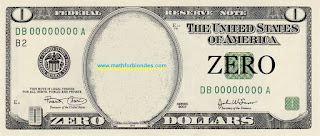Zero dollars

Another example from our life. We all go to the store and buy something. What is the buying process? This is the exchange of the money we have for the goods available in the store. The buying process itself can be compared to multiplication. If the buyer has money, and the store has goods, there are no problems. If the customer doesn't have the money or the store doesn't have the product, then you can't make the purchase. You wouldn’t go to the store with an empty wallet to hear from the seller that you can’t buy anything without money? This situation can be seen as an example of multiplication by zero.

Now consider the process of buying with "zero element". Imagine that you have a bill in your wallet that says "zero dollars". You go to the store with this bill and exchange it for a piece of paper with the inscription "zero goods". Technically, you made a purchase without having a cent in your pocket and without buying anything. Mathematicians tell us about a similar “multiplication by zero (zero element)”.

## Multiplication by zero is not possible

The substitution of concepts can change our logic beyond recognition. This modified logic forces us to look for multiplication by zero where it cannot be - in the results of multiplication. Since multiplication by zero does not occur, then you need to look not into the void (after all, there is no result of multiplication by zero), but into the initial conditions of multiplication. Two apples both lay and will remain lying, even after casting the spell "Apples, I multiply you by zero." Mathematically, this is written to the point of banality simply:

2*0=2*0

All this happens because our mathematicians have not learned to adequately describe reality with the help of mathematics. If you want to look at multiplication examples taken from real life, then you can do it here.

We will consider multiplication by zero in geometry and physics in more detail, to be continued.

P.S. What should you do? Remember that zero is not a number. And when it comes to zero in mathematics, discard your logic and common sense and open the Holy Mathematical Scripture. What is written about the case you are interested in, then tell the mathematicians. You will not begin to assert in a theological seminary that there is no God. So I do not recommend arguing with mathematicians - this is fraught with serious consequences for you. When you become adults and mathematicians disappear from your life, then you can say what you think is right.